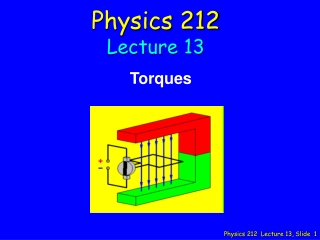DownloadDownload PresentationPhysics 212 Lecture 13

# Physics 212 Lecture 13

Download Presentation## Physics 212 Lecture 13

- - - - - - - - - - - - - - - - - - - - - - - - - - - E N D - - - - - - - - - - - - - - - - - - - - - - - - - - -
##### Presentation Transcript

1. Physics 212 Lecture 13 Torques

2. Main Point 1 First, we investigated the force on a straight section of a current carrying wire in a region containing a uniform magnetic field to determine that this force can be written as the current times the cross product of the length of the segment with the magnetic field. We then determined that if the wire were not straight, but rather curved, the same expression for the magnetic force holds, if we define the length vector to be the straight line that connects the ends of the segment. An important special case is the current loop, which since it begins and ends at the same point has a length vector of zero resulting in a zero net force on the loop.

3. Main Point 2 Second, we introduced an important new quantity m, the magnetic dipole moment of a current loop, which we used to quantitatively describe the interactions of the loop with a uniform magnetic field. m was defined to be a vector whose direction is perpendicular to the plane of the loop in a sense determined by yet another right hand rule, and whose magnitude was equal to the product of the current with the area of the loop. We determined that the torque exerted on the loop could be written simply as the cross product of m with the uniform magnetic field B.

4. Main Point 3 Finally, we defined the potential energy of a current loop in a uniform B field to be the negative of the work done by the magnetic field to change the orientation of m (mu) with respect to B. Choosing the zero of potential energy to be the position of maximum torque, we found we could write this potential energy U simply as minus the dot product of m with B.

5. Last Time: This Time: z y F B I x

6. ACT 08

7. Checkpoint 1a A B C 13

8. Checkpoint 1b y x • In which direction will the loop rotate? • (assume the z axis is out of the page) • Around the x axis • Around the y axis • Around the z axis • It will not rotate 15

9. Checkpoint 1c y A B C D E 17

10. Checkpoint 2a Three different orientations of a magnetic dipole moment in a constant magnetic field are shown below. Which orientation results in the largest magnetic torque on the dipole? 24

11. Checkpoint 2b Three different orientations of a magnetic dipole moment in a constant magnetic field are shown below. Which orientation has the most potential energy? 30

12. Checkpoint 2c Three different orientations of a magnetic dipole moment in a constant magnetic field are shown below. In order to rotate a horizontal magnetic dipole to the three positions shown, which one requires the most work done by the magnetic field? qa fa qc B 30

13. Magnetic Dipole Moment Area vector Magnitude = Area Direction uses R.H.R. Magnetic Dipole moment

14. z turnsmtoward B y m B z x m y B x turnsmtoward B mmakes torque easy! The torque always wants to linemup with B !

15. Magnetic Field can do Work on Current m B From Physics 211: From Physics 212:

16. Calculation z A square loop of side a lies in the x-zplane with current I as shown. The loop can rotate about x axis without friction. A uniform field B points along the +z axis. Assume a, I, and B are known. How much does the potential energy of the system change as the coil moves from its initial position to its final position. z . B B 30˚ y y a I x final initial Conceptual Analysis Strategic Analysis